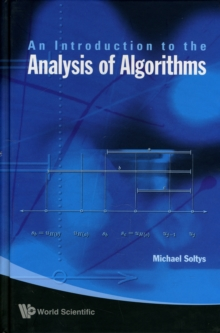Supporting your high street Find out how »
• My AccountIntroduction To The Analysis Of Algorithms, An Hardback

Description

This textbook covers the mathematical foundations of the analysis of algorithms.

The gist of the book is how to argue, without the burden of excessive formalism, that a given algorithm does what it is supposed to do.

The two key ideas of the proof of correctness, induction and invariance, are employed in the framework of pre/post-conditions and loop invariants.The algorithms considered are the basic and traditional algorithms of computer science, such as Greedy, Dynamic and Divide & Conquer.

In addition, two classes of algorithms that rarely make it into introductory textbooks are discussed.

Randomized algorithms, which are now ubiquitous because of their applications to cryptography; and Online algorithms, which are essential in fields as diverse as operating systems (caching, in particular) and stock-market predictions.This self-contained book is intended for undergraduate students in computer science and mathematics.

Information

• Format: Hardback
• Pages: 152 pages
• Publisher: World Scientific Publishing Co Pte Ltd
• Publication Date:
• Category: Maths for computer scientists
• ISBN: 9789814271400

Other Formats

£37.00

£31.15

on all orders

Pick up orders

from local bookshops

£54.00

£45.05